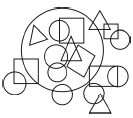# User Forum

Subject :NSO    Class : Class 4

How many different shapes are there in the figure?A2
B5
C3
D4

the correct answer should be 5 as there is a circle square triangle rectangle and semi-circle

## Ans 1:

Class : Class 4
How are we supposed to know itâs a rectangle when hidden by the circle ,bonne nuit

Class : Class 4
5

Class : Class 5

## Ans 4:

Class : Class 4
5 is the answer because there is even semi circle there with rectangle,square and triangle.

## Ans 5:

Class : Class 6
Can you tell the answer of this question

## Ans 6:

Class : Class 4
There is no semicircle.Answer is 4.

Class : Class 5

## Ans 8:

Class : Class 4
the answer can even be 'B' as semi-circle is there ,made by square

Class : Class 4
its d

Class : Class 5
5

Class : Class 3
ayurda 4 d 4

## Ans 12:

Class : Class 4
How 5? answer is 4The shapes are:circle,triangle,rectangle and square

## Ans 13:

Class : Class 4
55555555555555555555555555555555555555555 is the ans